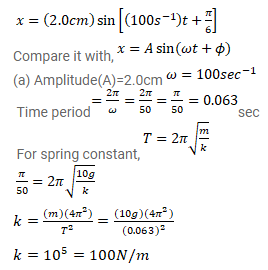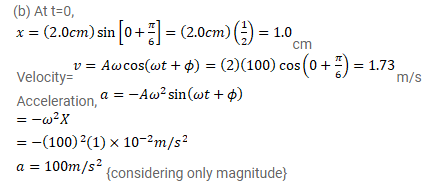# A particle having mass 10g oscillates according to the equation

Question:

A particle having mass $10 \mathrm{~g}$ oscillates according to the equation $x=(2.0 \mathrm{~cm}) \sin \left[\left(100 \mathrm{~s}^{-1}\right) t+\frac{\pi}{6}\right]$.

Find (a) the amplitude, the time period and the spring constant (b) the position, the velocity and the acceleration at $t=0$.

Solution: